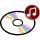Dr. S. R. Lasker Library Online Catalogue

Normal view

# Introductory econometrics : using Monte Carlo simulation with Microsoft Excel / Humberto Barreto, Frank M. Howland.

Material type:TextLanguage: English Publication details: 2006. Description: xxiii, 774 p. : ill. ; 26 cmISBN: 9780521843195; 0521843197DDC classification: 330.01518282 LOC classification: HB139 | .B376 2006Online resources: Worldcat details | Ebook Fulltext
Contents:
1. Introduction; Part I. Description: 2. Correlation; 3. Pivot tables; 4. Computing regression; 5. Interpreting regression; 6. Functional form; 7. Multivariate regression; 8. Dummy variables; Part II. Inference: 9. Monte Carlo simulation; 10. Inferential statistics review; 11. Measurement box model; 12. Comparing two populations; 13. The classical econometric model; 14. The Gauss Markov theorem; 15. Understanding the standard error; 16. Hypothesis testing and confidence intervals; 17. F tests; 18. Omitted variable bias; 19. Heteroskedasticity; 20. Autocorrelation; 21. The series topics; 22. Dummy dependent variables; 23. Bootstrap; 24. Simultaneous equations. TOC
Tags from this library: No tags from this library for this title.
Star ratingsAverage rating: 0.0 (0 votes)
Holdings
Item type Current library Collection Call number Copy number Status Date due Barcode Item holdsE-Book
E-book
Non-fiction 330.01518282 BAI 2006 (Browse shelf(Opens below)) Not for loanText
Reserve Section
Non-fiction 330.01518282 BAI 2006 (Browse shelf(Opens below)) C-1 Not For Loan 19627CDs & DVDs
Audio Visual
Non-fiction 330.01518282 BAI 2006 (Browse shelf(Opens below)) C-1 Available CD-1165
Total holds: 0

Includes bibliographical references and index.

1. Introduction; Part I. Description: 2. Correlation; 3. Pivot tables; 4. Computing regression; 5. Interpreting regression; 6. Functional form; 7. Multivariate regression; 8. Dummy variables; Part II. Inference: 9. Monte Carlo simulation; 10. Inferential statistics review; 11. Measurement box model; 12. Comparing two populations; 13. The classical econometric model; 14. The Gauss Markov theorem; 15. Understanding the standard error; 16. Hypothesis testing and confidence intervals; 17. F tests; 18. Omitted variable bias; 19. Heteroskedasticity; 20. Autocorrelation; 21. The series topics; 22. Dummy dependent variables; 23. Bootstrap; 24. Simultaneous equations. TOC

Economics

Shamima Yeasmin

There are no comments on this title.